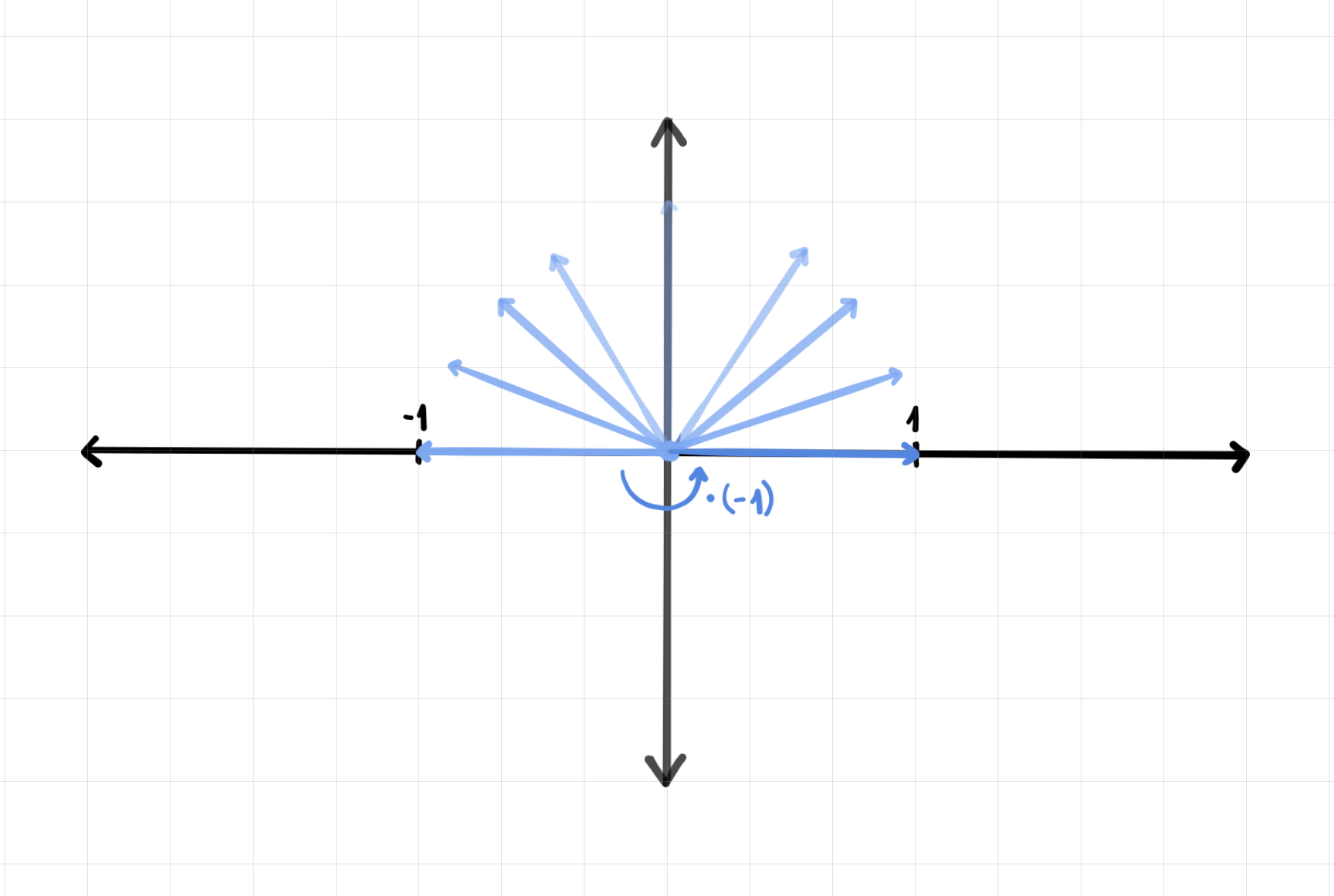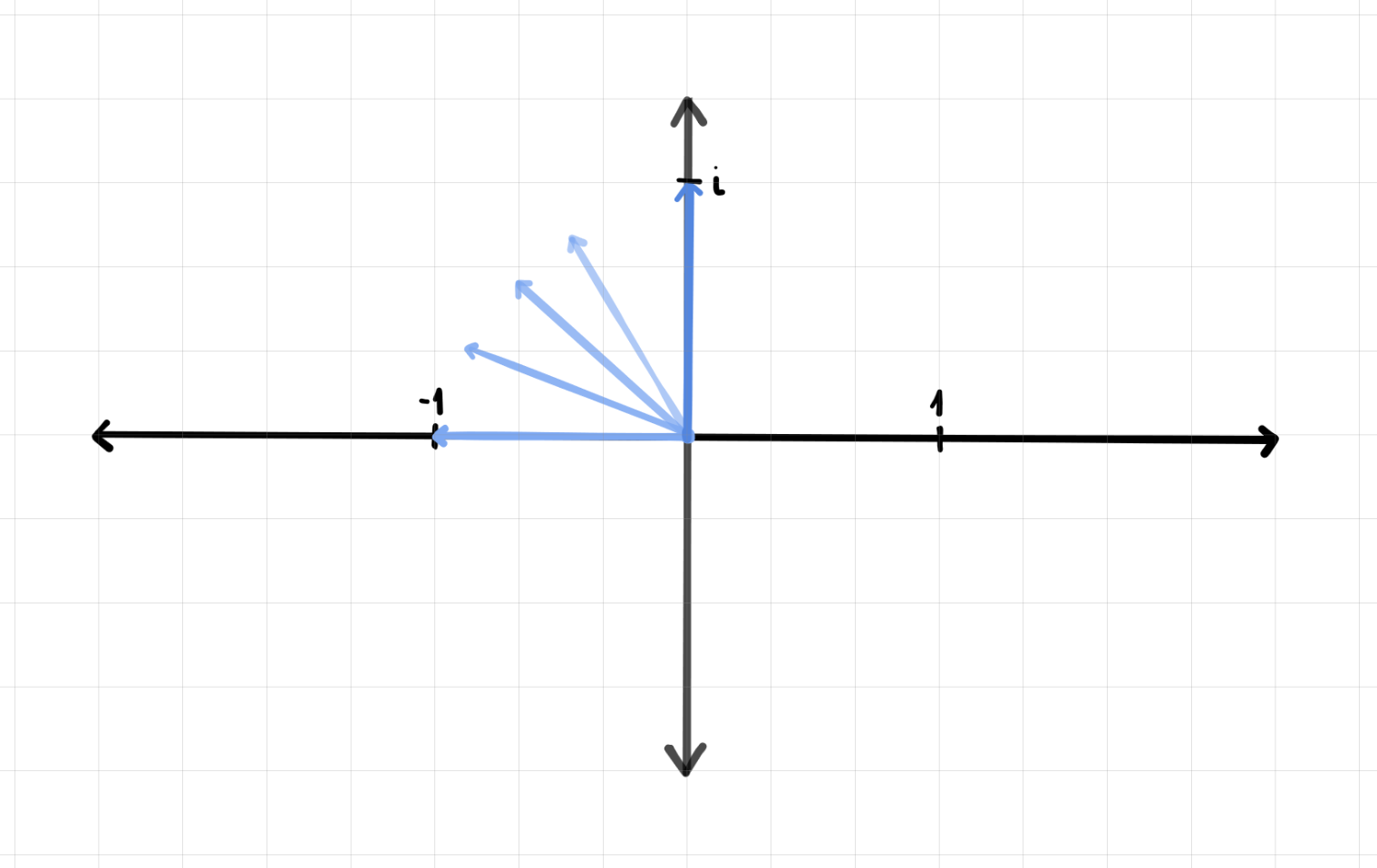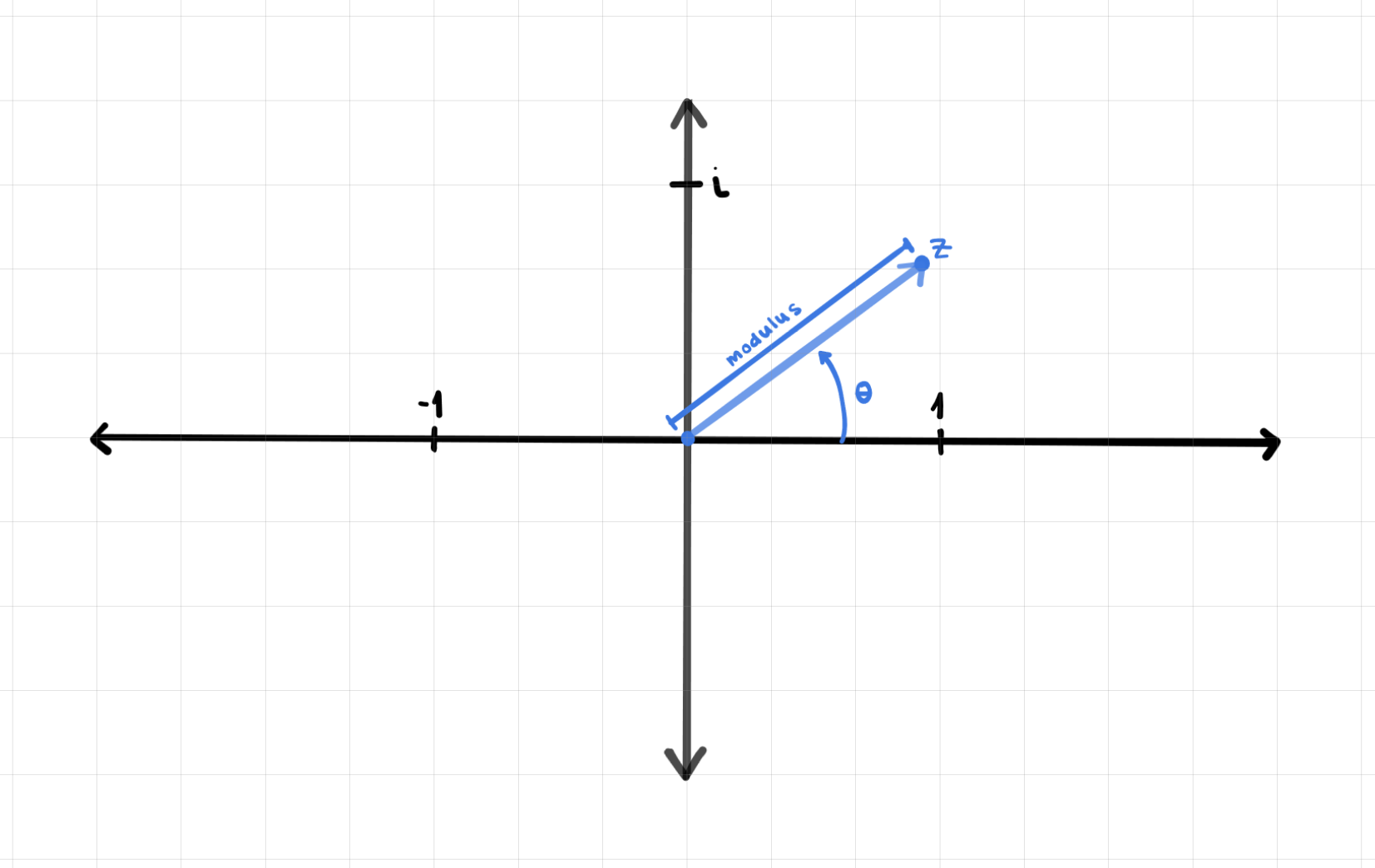# Unit One: Linear Algebra and Complex Numbers Crash Course

1.1 Introductions to Textbooks
Takeaway
I’m using two main textbooks for this independent study:  The Mathematics of Quantum Mechanics and Quantum Theory, Groups, and Representations. The Mathematics of Quantum Mechanics is a textbook aimed at high school students and therefore provides a great simplistic baseline, although it doesn’t go into much depth about any particular topic and only covers a couple topics. Quantum Theory, Groups, and Representations, on the other hand, is written based on a college physics course and is much heavier on the technical aspect side of things.

Quotes from The Mathematics of Quantum Mechanics I found compelling
“Classical physics is only an approximation”
“Quantum mechanics is the most accurate theory ever developed by mankind. Every attempt to prove it wrong has failed miserably.”

Topics from Quantum Theory, Groups, and Representations to future explore
* Information theory

1.2 Complex Numbers
Takeaway
Complex numbers are at the center of quantum mathematics, and unlike classical physics, complex numbers cannot be split apart: imaginary and real parts must be regarded together. But before we get ahead of ourselves, let’s backtrack to the basics of complex numbers.

Real numbers are the numbers we almost always use in our day to day lives, ex. 7, -23, pi. All real numbers must be positive when squared.

Imaginary numbers, on the other hand, must be negative when squared. Another way to think about this is that imaginary numbers are the square roots of positive numbers. The “imaginary number unit” is i, which is the square root of -1. This is extremely hard to conceptualize because it goes against our perception of how numbers work. What multiplied by itself is negative? Let’s instead think of imaginary numbers as rotations.

If i is the square root of -1, then `i2 = -1`. `-1 x -1 = 1`. So far so good. This is illustrated below as a rotation.So, if multiplying by i rotates the arrow, and multiplying by i2 rotates the arrow from -1 to 1, multiplying by a single i will rotate the arrow halfway, as pictured below.An interesting development emerges! Imaginary numbers (we were using i in this example) act almost like a second dimension and axis. The numbers we use are actually two dimensional; we just tend to tend to use only the classic number line.

The name of these two dimensional numbers is “complex numbers“. The formal definition of complex numbers is “any number written in from `z = a + bi`, where a and b are real numbers.” a is called the real part of the complex number – Re(z), and b is the imaginary part – Im(z).

The graph above is called a “complex plane“, which is used to visualize complex numbers using the x-axis to denote the real part, and the y-axis to denote the imaginary part. Complex numbers, `z = a + bi`, can be represented as a point on the complex plane where a is the x value and b is the y value.

Like with real numbers, there’s a variety of operations that can be preformed on complex numbers. The summary of properties can be found in the notes below, but the general rule is that real and imaginary numbers cannot be combined. Real numbers can be combined with real numbers, and imaginary numbers can be combined with imaginary numbers, but they cannot be inter-combined.

There’s a couple of other definitions that form the foundation of complex numbers:
* Complex Conjugate: the complex conjugate of a complex number `a + bi `is `a - bi`.
* Modulus: the modulus of a complex number `a + bi` is the square root of `a2 + b2`. This number will always be real and positive. The modulus represents the distance from the origin to the complex number on the complex plane.

Complex numbers, however, when represented as `a + bi` can be hard to work with. Thankfully, the polar form of the equation offers some help. The polar form deals with the angle (theta) and length (modulus) of the complex number, as shown below, instead of representing the number in terms of x and y values. Theta is known as the argument of the complex number.If we use this polar form, a can be expressed as the `(modulus of z) *cos(theta)` and b can be expressed as `(modulus of z) *sin(theta)`. Therefore, `z = a + bi` can be rewritten as `z = (modulus of z) * [cos(theta) + sin(theta)i]`. This is immensely helpful because of Euler’s Formula: `ei(theta) = cos(theta) + sin(theta)i`. Putting all of this together, `z = (modulus of z)ei(theta)`. A summary of the properties of complex numbers when in this form can be found in the notes.

And that’s it for the fundamentals of complex numbers! To return to the beginning, complex numbers are so core to quantum mechanics because, unlike in classical physics, the components of the complex number cannot be separated and treated independently. To quote “Why are complex numbers needed in quantum mechanics?” (see resource list below), “Quantum mechanics deals with complex quantities of a special kind, which cannot be split into real and imaginary parts”. For complex numbers this is great news. For a few hundred years, they were nothing more than a mind bending mathematical party trick. Now, they are fundamental in the fabric of the universe.

Resources
* The Mathematics of Quantum Mechanics
* Why are complex numbers needed in quantum mechanics?
* Necessity of complex numbers
* Illinois Course Phys 580

Topics to further explore
* Wave function and quantum state vectors
* Wave formulation
* Stern-Gerlach experiments

Notes

Video Lesson

1.3 Linear Algebra Basics
Takeaway

IdyStudy__Quantum_Mechanics

Resources
* Chapter Two: Linear Algebra in The Mathematics of Quantum Mechanics
* UCSB Math/CS 120
* Matrix Transformations of Khan Academy’s Linear Algebra Course
* Chapter Four: Linear Algebra Review, Unitary and Orthogonal Groups in Quantum Theory, Groups, and Representations
* Eigenvectors and eigenvalues of 3Blue1Brown’s Essence of Linear Algebra series

Notes

1.3-Linear-Algebra-Basics-Notes